# Strength Envelope for a Slope Application in Highly Disturbed Rock

## 1.0 Introduction

For this tutorial we consider a rock mass with the same basic parameters as the previous tutorial examples, but in a highly disturbed slope of 100 meters height, with a disturbance factor D = 1.

Finished Product:

The finished product of this tutorial can be found in the Tutorial 2.rsdata data file. All tutorial files installed with RSData can be accessed by selecting File > Recent Folders > Tutorials Folder from the RSData main menu.

## 2.0 Creating the model

1. Open up the RSData program.
2. Select the Rock Template and click OK.
3.4. Click on the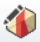Define Material Models icon in the toolbar.
5. By default, the Define by field should already have GSI, MI, d selected. This selection allows you to use some of the GSI inputs directly in the Strength tab. Other fields though can only be viewed in the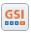GSI calculator. To access the GSI calculator you can change the Define by input to mb, s, a and the GSI calculator icon will become active. For this tutorial we will leave GSI, Mi, d selected.

For this example we will use the same inputs as in Tutorial 1:

• UCS of intact rock (intact) (MPa) = 75
• GSI = 55
• mi (aka Intact Rock Constant mi) = 17
6. Next to the d (Disturbance factor) input click on the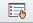picker button.
7.8. Select Slopes as the application.
9. Select D=1.0 Production Blasting from the list as follows.
10. Click OK to close the dialog.
11. Note that both Poor Blasting and Production Blasting in slope result with D = 1.0.

The dialog should look as follows: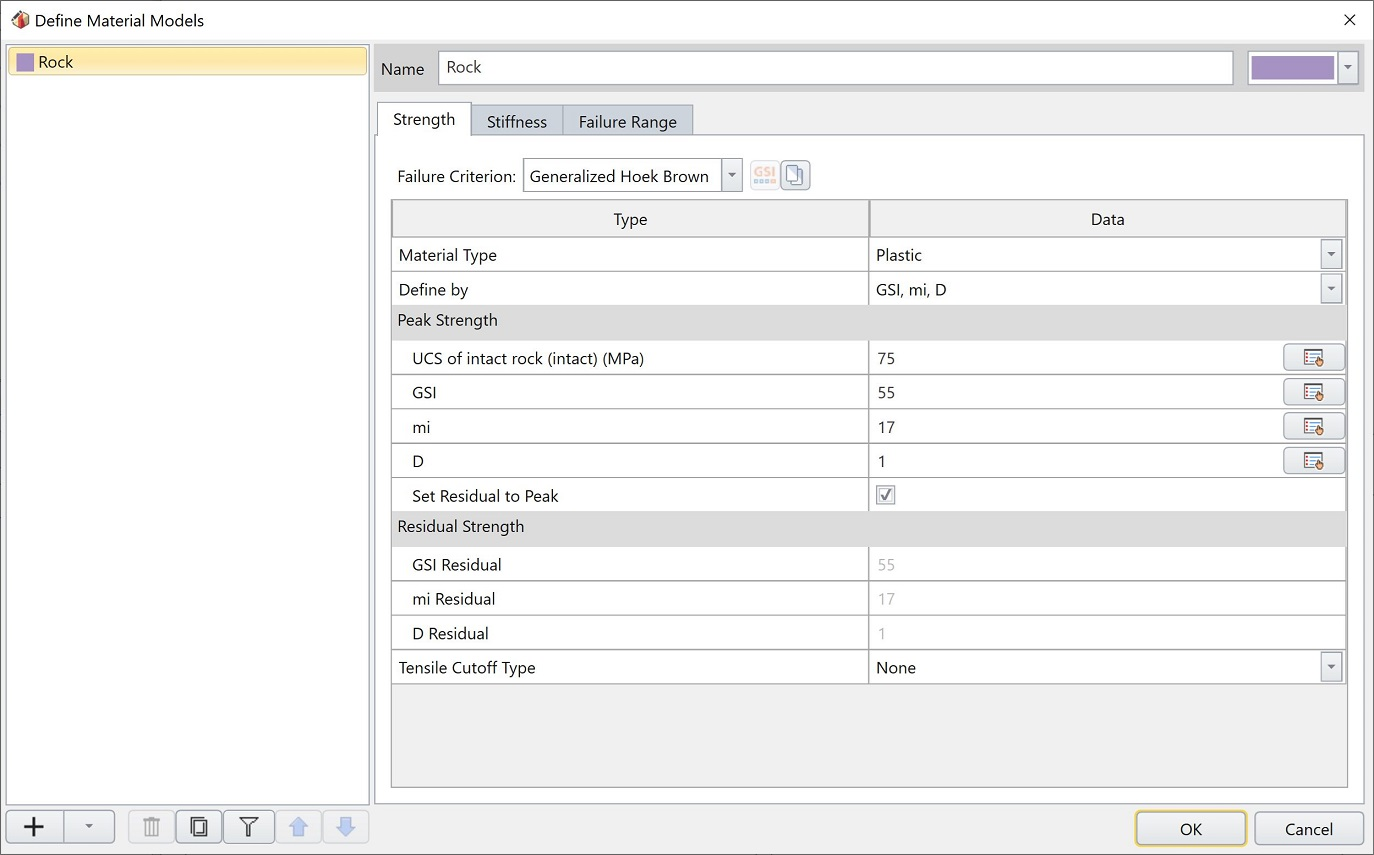12. Select the Failure Range tab. Change the application to Slopes and enter the following data to determine the failure Envelope Range (sig3max):
• Unit weight = 0.027
• Slope Height = 100
• Number of Points = 20013. Click OK to save and close the Define Material Model dialog.

## 3.0 Results

The Normal vs. Shear Stress Plot is updated as follows:Select thearrow on the right side of the screen to open the results panel. Then go to the Visibility Tree and select Material Model (Rock).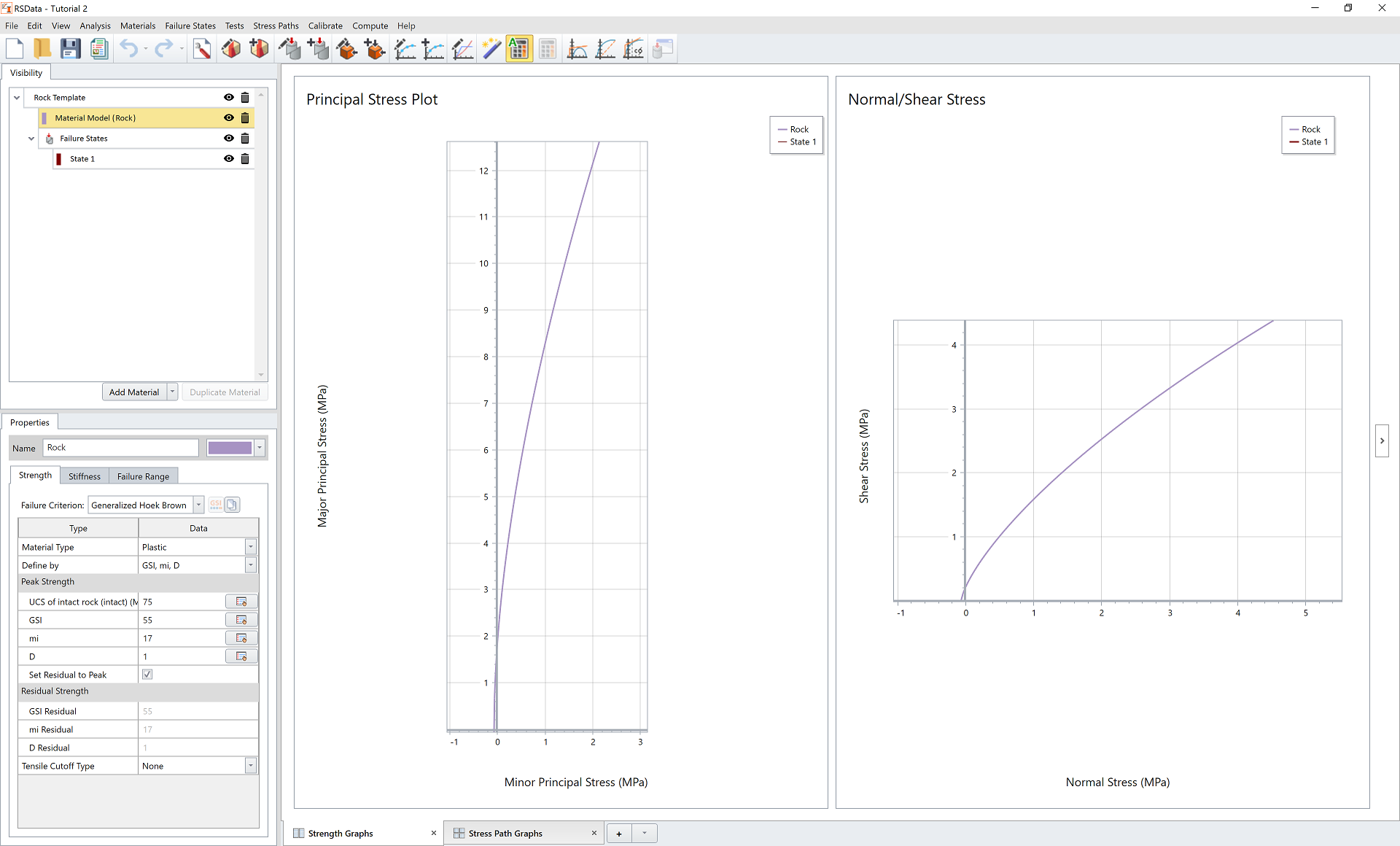The resulting outputs and equivalent Mohr-Coulomb parameters are calculated and displayed in the data table as shown below.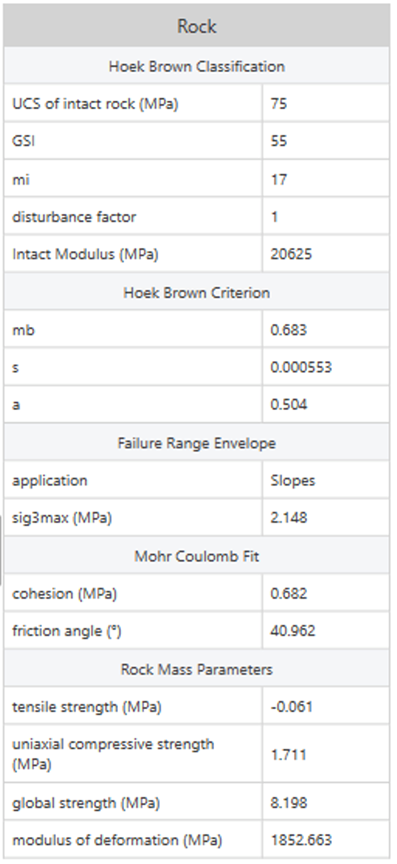Compare the Rock Mass Parameters calculated in Tutorial 1 and Tutorial 2. The parameter mainly affected by the factor D is the modulus of deformation (Em or Young’s modulus), where the Em has significantly been reduced in Tutorial 2 (1852 MPa) compared to that in Tutorial 1 (8420 MPa).This demonstrates the significant effect which the disturbance factor D can have on the calculated rock mass strength.

For information about the Disturbance Factor, see the following reference (section 7: Estimation of Disturbance Factor D):

• Hoek, E., Carranza-Torres, C. and Corkum, B., (2002) Hoek-Brown Failure Criterion – 2002 Edition. 5th North American Rock Mechanics Symposium and 17th Tunneling Association of Canada Conference: NARMS-TAC, 2002, pp. 267-271.

See the Generalized Hoek-Brown help topic for a link to this paper.